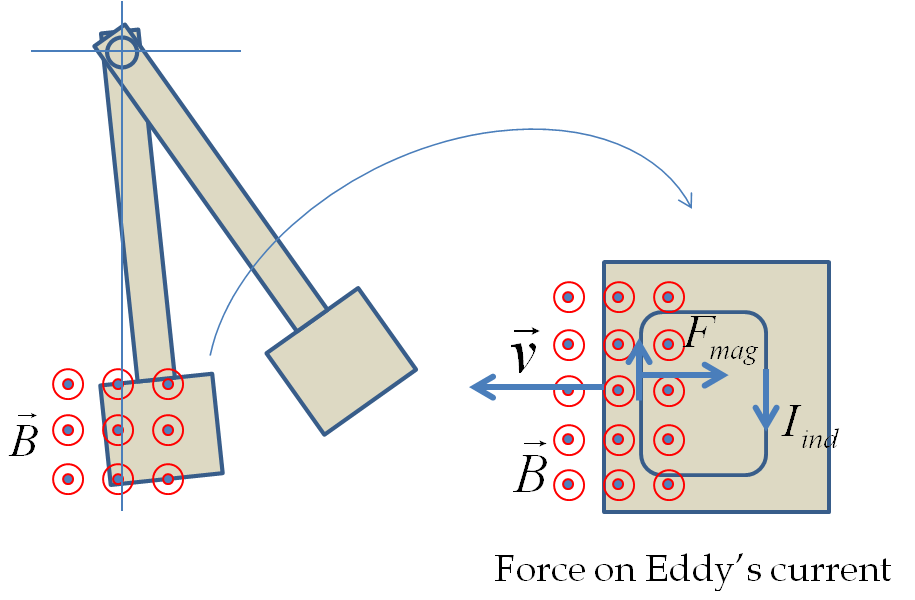## Section41.6Eddy's Current

A moving conductor experiences a retarding force when moving in a region of magnetic field. This effect is called magnetic braking. It has a number of applications. Magnetic braking can be understood on the basis of Faraday's law as follows.

A continuous conductor provides infinitely many loops in which electrons can freely move. Therefore, if you place a conductor in a magnetic field such that the magnetic flux through any part of the conductor changes, currents would be induced in the body of the conductor. These currents are called Eddy's currents.

The existence of Eddy's current in conductors is responsible for magnetic braking. The changing magnetic flux through a conductor induces Eddy's currents inside the conductor, which are then subject to magnetic force from the magnetic field.

Illustration of magnetic braking. Consider a metal bar suspended at one end as shown in Figure 41.6.1. When the bar is released, it goes between the poles of a magnet. Suppose, the magnetic poles are arranged so that the magnetic field in the plane of the metal bar's motion is pointed out-of-page.

When the bar enters the non-zero field region, a clockwise current is induced inside the bar. Some of these loops are large enough that magnetic field acts only on the left end of the loop. This gives a net force on the bar pointed in the opposite direction of velocity as shown in Figure 41.6.1. Since acceleration is in the opposite direction to velocity, the bar slows down when entering a region of non-zero magnetic field. The kinetic energy of the bar is converted into thermal energy by current inside the conductor.Figure 41.6.1. When the moving metal enters a region of a non-zero magnetic field, the magnetic flux through loops in the body of the conductor changes. One loop is shown in the figure to the right. The changing magnetic flux causes induced current loops in the body of the conductor called Eddy's current. If the loop is large enough, then the induced current at different ends of the loop will experience different magnetic forces. A net magnetic force on larger loops decelerates the body and the body slows down as it enters a region of non-zero magnetic field. If slots are cut in the body to prevent large loops, then the braking does not occur.

If you cut slots in the bar so that large loops are not possible as shown in Figure 41.6.2, then you prevent large eddy loops. So, although currents will be induced in tiny loops in the metal, there will be no magnetic braking.